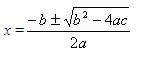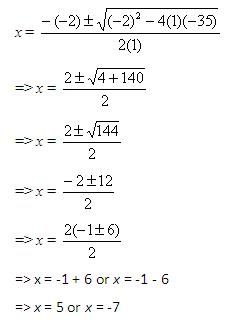## Feb 15, 2010

The solution of a quadratic equation in the standard form ax2 + bx + c = 0, where a, b, and c are real numbers and a 0, can be find using the quadratic formula given below.Let us work out a few examples.
Example 1:
Use quadratic formula to solve x2 - 2x - 35 = 0.
Solution:
Step 1:
Given equation is x2 - 2x - 35 = 0.
Here, a = 1, b = -2, and c = -35.Step 2:
Substitute the values of a, b, and c in the quadratic formula to find the value of x.Step 3:
So, the solution set is {5, -7}.
Example 2:
Use quadratic formula to solve 4x2 + 2x = 17.
Solution:
Step 1:
Given equation is 4x2 + 2x = 17.
It can be written as 4x2 + 2x - 17 = 0. The equation is in the form of ax2 + bx + c = 0, where a = 4, b = 2, and c = -17.
Step 2:Substitute the values of a, b, and c in the quadratic formula to find the value of x.Step 3:
So, the solutions are 1.83 and -2.33.
Practice questions: